# KVPY-SA 2019 Maths Paper With Solutions

KVPY-SA 2019 Maths Question Paper with Solutions is available here. It will keep the students in good stead as the exam approaches. It helps the candidates to get an idea on the weightage given to various topics. Candidates can download the previous years’ question papers of KVPY to understand the question paper pattern. Practising the previous year papers is the best tool for the preparation of the competitive exam KVPY, in terms of solving questions faster and in learning time management. Solving these papers with solutions will help the students in knowing the questions that are frequently asked in the examination.

### KVPY-SA 2019 - Maths

Question 1: Let ABC be an equilateral triangle with side length a. Let R and r denote the radii of the circumcircle and the incircle of triangle ABC respectively. Then, as a function of a, the ratio R / r

1. a. strictly increases
2. b. strictly decreases
3. c. remains constant
4. d. strictly increases for a < 1 and strictly decreases for a > 1

Solution: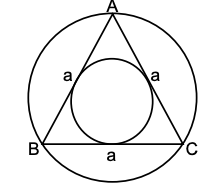Given

△ABC is equilateral triangle

Side = a

(a = b = c) = a …………..(i)

Area of equilateral triangle (△) = (√3 / 4) a2

Radii of the circumcircle of the triangle

(R) = [abc] / [4 × Area of equilateral triangle] = (abc / 4△) ----- (ii)

Radii of the incircle of the triangle

(r) = Area of equilateral triangle / Semiperimeter of the triangle = (△ / s) ---- (iii)

Now,

(R / r) = (abc / 4△) * (s / △) = [a . a . a. s] / [4△2] {from equation (1)}

(R / r) = {a3 [a + b + c] / 2} / {4 [(√3 / 4) a2]} {because s = [a + b + c] / 2}

(R / r) = {a3 [3a / 2]} / [(3 / 4)a4] {from equation (1)}

(R / r) = 2 (constant)

Question 2: Let b be a non-zero real number. Suppose the quadratic equation 2x2 + bx + (1 / b) = 0 has two distinct real roots. Then

1. a. b + (1 / b) > (5 / 2)
2. b. b + (1 / b) < (5 / 2)
3. c. b2 – 3b > – 2
4. d. b2 + (1 / b2) < 4

Solution:

The given equation is 2x2 + bx + (1 / b) = 0, comparing it with Ax2 + Bx + C = 0. We get A = 2, B = b, C = 1 / b

Now, it is given that roots are real. So,

Discriminant > 0

B2 - 4AC > 0

b2 - 4(2) (1 / b) > 0

b2 - (8 / b) > 0

{[b3 - (2)3] / b} > 0 {P3 – Q3 = (P – Q) (P2 + PQ + Q2)}

[(b - 2) (b2 + 2b + 4)] / b > 0

b2 + 2b + 4 always positive (D < 0 ⇒ (2)2 – 16 < 0)

[b - 2] / b > 0

b – 2 > 0, b > 0 OR b – 2 < 0, b < 0

b > 2, b < 0

b ∈ (-∞, 0) ⋃ (2, ∞)

Check for the options:

(A) b + (1 / b) > (5 / 2)

b > 2 satisfied but b < 0 not satisfied

(B) b + (1 / b) < (5 / 2)

b < 0 satisfied but b > 2 not satisfied

(C) b2 – 3b > – 2

b2 – 3b + 2 > 0

b2 – 2b – b + 2 > 0

(b – 2) (b – 1) > 0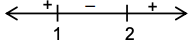b ∈ (-∞, 1) ⋃ (2, ∞)

Satisfied both conditions.

(D) b2 + (1 / b2) < 4

b < 0 & b > 2 not satisfied both condition

Question 3: Let p(x) = x2 + ax + b have two distinct real roots, where a, b are real numbers. Define g(x) = p(x3) for all real numbers x. Then which of the following statements are true?

I. g has exactly two distinct real roots

II. g can have more than two distinct real roots

III. There exists a real number such that g (x) ≥ α for all real x

1. a. Only I
2. b. Only I and III
3. c. Only II
4. d. Only II and III

Solution:

Given P(x) = x2 + ax + b {roots are α, β}

Roots are real so D > 0 ……….(i)

P(x) = x2 – (–a) x + b

P(x) = x2 – (α + β) x + αβ {Sum of roots = –a, Product of roots = b}

P(x) = x(x – α) – β (x – α)

P(x) = (x – α) (x – β)

Replacing x → x3

P(x3) = (x3 – α) (x3 – β)

g(x) = (x3 – α) (x3 – β) {Given P(x3) = g(x)}

Let α = a13 and β = b13

g(x) = (x3 – a13)(x3 – b13) {P3 – Q3 = (P – Q) (P2 + PQ + Q2)}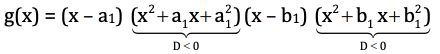g(x) = 0 for exactly two real values of x,

g(x) = x6 + ax3 + b

Since, g(x) is even degree polynomial and leading coefficient is greater than zero So, g (x) ≥ α for all real x.

Question 4: Let, n ≥ 1, be an arithmetic progression with first term 2 and common difference 4. Let Mn be the average of the first n terms. Then the sum ∑n=110 Mn =

1. a. 110
2. b. 335
3. c. 770
4. d. 1100

Solution:

Given

First term a1 = 2

Common difference (d) = 4

Mn = Average of first n terms

Mn = [sum of first n terms] / n = Sn / n

= {(n / 2) (2a + [n - 1] d)} / n

= n [2 (2) + (n - 1) 4] / 2n

= [4 + 4n - 4] / 2

= 4n / 2

= 2n

Now,

n=110 Mn = ∑n=110 2n

= 2 [1 + 2 + 3 + …… + 9 + 10]

= 2{[(10) (10 + 1)] / 2} Sum of first n natural numbers = n [n + 1] / 2}

= 10 × 11

= 110

Question 5: In a triangle, ABC, ∠BAC = 90°; AD is the altitude from A onto BC. Draw DE perpendicular to AC and DF perpendicular to AB. Suppose AB = 15 and BC = 25. Then the length of EF is

1. a. 12
2. b. 0
3. c. 5 √3
4. d. 5 √5

Solution: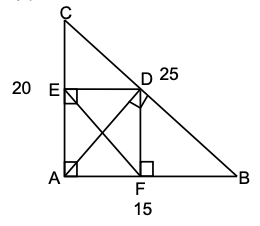Given ∠BAC = 90°

AD ⊥ BC , DE ⊥ AC and DF ⊥ AB

AB = 15 and BC = 25

EDFA is rectangle

AD = EF {Diagonals of a rectangle are equal}

Now, ABC is a right angle triangle.

(AB)2 + (AC)2 = (BC)2 {By Pythagoras theorem}

(15)2 + AC2 = (25)2

225 + (AC)2 = 625

(AC)2 = 625 – 225

AC = √400

AC = 20

Let BD = 25 – x

So CD = x

(AD)2 = (20)2 – x2 …………….(1)

(AD)2 = (15)2 – (25 – x)2 ……………..(2)

Equation (2) = Equation (1)

(15)2 – (25 – x)2 = (20)2 – x 2

225 – (625 – 50x + x2) = 400 – x2 { (A + B)2 = A2 + B2 + 2AB)

225 – 625 + 50x – x2 = 400 – x2

50 x = 800

x = 16

CD = 16

From equation (1)

Question 6: The sides a, b, c of a triangle satisfy the relations c2 = 2ab and a2 + c2 = 3b2. Then the measure of ∠BAC, in degrees, is

1. a. 30
2. b. 45
3. c. 60
4. d. 90

Solution:

In △ABC

Sides a, b, c

Given:

c2 = 2ab ……….(1)

a2 + c2 = 3b2 ……….(2)

a2 + 2ab = 3b2 {from equation (1)}

a2 + 2ab + b2 = 4b2 { (A + B)2 = A2 + B2 + 2AB)

(a + b)2 = 4b2

a + b = ± 2b

Sides are always positive and sum of two sides are positive.

So, sum of two sides are not negative

a + b = + 2b

a = b

Substitute a = b in equation (1) we get

c2 = 2a(a)

c2 = 2a2

c = √2a

We can see c2 = a2 + b2 {△ABC is a right angle triangle.}

c2 = a2 + a2 { a = b}

c2 = 2a2

c = (√2) a

We can see △ABC is a right-angled triangle and c is the hypotenuse.

∠C = 90°

∠A + ∠B = 90°

∠A + ∠A = 90° {Base angles of an equal side also an equal}

∠A = 45° = ∠B

∠BAC = 45°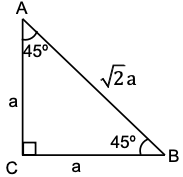Question 7: Let N be the least positive integer such that whenever a non-zero digit c is written after the last digit of N, the resulting number is divisible by c. Then the sum of the digits of N is

1. a. 9
2. b. 18
3. c. 27
4. d. 36

Solution:

Sum of digit of N should be divisible by 9.

In this case, the resulting number will always be divisible if C = 1, 2, 3, 5, 6 and 9 To make the resulting number divisible by 4, 7 and 8 as well N should be a multiple of LCM of (4, 7, 8) i.e. 56

A smallest positive integer which is multiple of 56 and whose sum of digits is a multiple of 9 is 504.

N will be 504

Sum of digits = 9

Question 8: Let x1, x2,....., x11 be 11 distinct positive integers. If we replace the largest of these integers by the median of the other 10 integers, then

1. a. the median remains the same
2. b. the mean increases
3. c. the median decreases
4. d. the mean remains the same

Solution:

Let x1, x2,....., x11 are distinct positive integers suppose the increasing order of the numbers are x1, x2, x3, x4, x5, x6, x7, x8, x9, x10, x11

And x11 is the largest number

And the median is x6

Now, the median of first 10 numbers is [x5 + x6] / 2 = t (let) 2 and we have to replace largest number (x11) by the median of first 10 No.’s i.e. (t)

So, the new increasing order will be

x1, x2, x3, x4, x5, x6, x7, x8, x9, x10

And new median will be t i.e. [x5 + x6] / 2 which is lesser than the median of first eleven No. ‘x’6 so median decreases.

Question 9: The number of cubic polynomials P(x) satisfying P(1) = 2, P(2) = 4, P (3) = 6, P (4) = 8 is

1. a. 0
2. b. 1
3. c. more than one but finitely many
4. d. infinitely many

Solution:

Given P(1) = 2, P(2) = 4, P(3) = 6, P(4) = 8

Let a cubic polynomial p(x) = ax3 + bx2 + cx + d

Now

P(1) = a(1)3 + b(1) + c(1) + d

2 = a + b + c + d ……..(1)

P(2) = a(2)3 + b(2)2 + C(2) + d

4 = 8a + 4b +2c +d ….….(2)

P(3) = a(3)3 + b(3)2 + c(3) + d

6 = 27a + 9 b + 3c + d ..……..(3)

P(4) = a(4)3 + b(4)2 + C(4) + d

8 = 64a + 16b + 4c + d …….. (4)

Subtracting equation (1) from (2) we get,

8a + 4b + 2c + d – a + b + c + d = 4 – 2 7a + 3b + c = 2 ………(5)

Subtracting equation (2) from (3) we get

27a + 9 b + 3c + d – 8a + 4b + 2c +d = 6 – 4 19 a + 5 b + c = 2 …..…..(6) Subtracting equation (3) from (4) we get

64a + 16b + 4c + d – 27a + 9b + 3c + d = 8 – 6 37a + 7b + c = 2 ……….(7) Subtracting equation (5) from (6) we get

19 a + 5 b + c – 7a + 3b + c = 2 – 2 12a + 2b = 0 ………..(8)

Subtracting equation (6) from (7) we get

37a + 7b + c – 19 a + 5 b + c = 2 – 2 18a + 2b = 0 …………(9)

Subtracting equation (8) from (9) we get

18a + 2b – 12a + 2b = 0

6a = 0

a = 0

If a = 0 then polynomial will be

P(x) = (0)x3 + bx2 + cx + d

P(x) = b(x)2 + cx + d

i.e. there is no cubic polynomial possible.

Question 10: A two-digit number ab is called almost prime if one obtains a two-digit prime number by changing at most one of its digits a and b. (For example, 18 is an almost prime number because 13 is a prime number). Then the number of almost prime two-digit numbers is

1. a. 56
2. b. 75
3. c. 87
4. d. 90

Solution:

Total numbers of two-digit number = 90

In each row from 10 to 19, 20 to 29, 30 to 39,……….,90 to 99

Has at least one prime number so each number can be reduced into prime number by changing at most one digit.

Question 11: Let P be an interior point of a convex quadrilateral ABCD and K, L, M, N be the midpoints of AB, BC, CD, DA respectively. If Area (PKAN) = 25, Area (PLBK) = 36, and Area (PMDN) = 41, then Area (PLCM) is

1. a. 20
2. b. 29
3. c. 52
4. d. 54

Solution: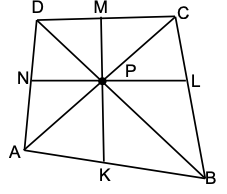K, L, M, N be the midpoints of AB, BC, CD, DA.

Δ1 = Area of △PAK = area of △PBK {AK = BK}

Similarly Ar △PBL = Ar △PCL = Δ2

Ar △PMC = Ar △PMD = Δ3

Ar △PMN = Ar △PNA = Δ4

Given,

Ar(PKAN) = Δ1 + Δ4 = 25 ………(1)

Ar(PLBK) = Δ1 + Δ2 = 36 ………(2)

Ar(PMDN) = Δ4 + Δ3 = 41 ………(3)

Now, Equation (2) + equation (3) – equation (1)

Δ1 + Δ2 + Δ4 + Δ3 – Δ1 – Δ4 = 36 + 41 – 25

Δ2 + Δ3 = 52

Ar(PLCM) = 52

Question 12: The number of non-negative integer solutions of the equations 6x + 4y + z = 200 and x + y + z = 100 is

1. a. 3
2. b. 5
3. c. 7
4. d. infinite

Solution:

Given equation:

6x + 4y + z = 200 ……(1)

x + y + z = 100 ……(2)

Subtracting equation (1) from (2) we get,

5x + 3 y = 100 ……(3)

Number of ordered pair (x, y) satisfying equation (3) is (2, 30), (5, 25), (8, 20), (11, 15), (14, 10), (17, 5), (20, 0)

(x, y, z) ∈ {(2, 30, 68), (5, 25, 70), (8, 20, 72), (11, 15, 74), (14, 10, 76), (17, 5, 78), (20, 0, 80)}

Number of non negative integral solution = 7

Question 13: Let N1 = 255 + 1 and N2 = 165. Then

1. a. N1 and N2 are coprime
2. b. the HCF (Highest Common Factor) of N1 and N2 is 55
3. c. the HCF of N1 and N2 is 11
4. d. the HCF of N1 and N2 is 33

Solution:

Given N1 = 255 + 1 ………(1)

N2 = 165 = 5 × 3 × 11

From equation (1)

N1 = (25)11 + 1

N1 = (32)11 + 1

N1 = (33 – 1)11 + 1 {Using Binomial expansion (x + y)n =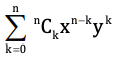}

N1= 11C0 (33)1111C1(33)10 + 11C2(33)9 – ………….+ 11C10(33) – 11C11 (33)0 + 1

N1 = 11C0 (33)1111C1(33)10 + ……………… + 11C10(33) – 1 + 1

N1 = 11C0 (33)1111C1(33)10 + ……………… + 11C10(33)

N1 is divisible by 33

Also, the units digit of N1 is 9

N1 is not divisible by 5

HCF of N1 and N2 = 33

Question 14: Let l > 0 be a real number, C denotes a circle with circumference l, and T denote a triangle with perimeter l. Then

1. a. given any positive real number α, we can choose C and T as above such that the ratio Area(C) / Area(T) is greater than α
2. b. Given any positive real number α, we can choose C and T as above such that the ratio Area(C) / Area(T) is less than α.
3. c. given any C and T as above, the ratio Area(C) / Area(T) is independent of C and T
4. d. there exist real numbers a and b such that for any circle C and triangle T as above, we must have a < Area(C) / Area T < b

Solution:

Let the radius of circle = r and sides of the triangle be a, b, c

Given that

Circumference of circle = l = perimeter of the triangle

2𝝿r = l = a + b + c ⇒ r = l / 2𝝿 ---- (1)

Area of circle = 𝝿r2 = l2 / 4𝝿 {from equation (1)}

Area of triangle (Δ) = √(s (s - a) (s - b) (s - c))

Where 2s = a + b + c = l {s is denoted by perimeter of triangle}

Using the concept of A.M. ≥ G.M.

AM ≥ GM

[(s - a) + (s - b) + (s - c)] / 3 ≥ [(s - a) + (s - b) + (s - c)]

(s - a) + (s - b) + (s - c) ≤ (s / 3)3

Taking a square root both sides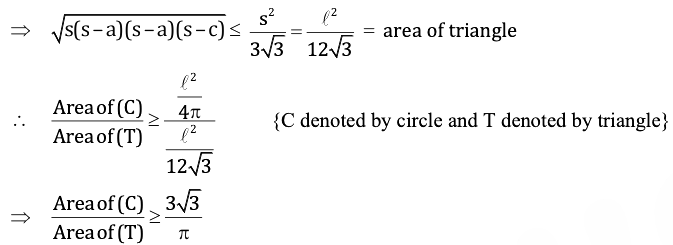Hence for given positive real number α we can choose C and T such that the ratio is greater than α.

Question 15: The number of three-digit numbers abc such that the arithmetic mean of b and c and the square of their geometric mean is equal is

1. a. 9
2. b. 18
3. c. 36
4. d. 54

Solution:

Given that

Arithmetic mean = (geometric mean)2

[b + c] / 2 = (√bc)2

[b + c] / 2 = bc

(b / 2) + (c / 2) - bc = 0

bc - (b / 2) - (c / 2) + (1 / 4) = 1 / 4

[b - (1 / 2)] [c - (1 / 2)] = 1 / 4

(2b – 1) (2c – 1) = 1

(2b – 1) = 1 and (2c – 1) = 1 or (2b – 1) = – 1 and (2c – 1) = – 1

b = 1 and c = 1 or b = 0 and c = 0

No’s can be of form a11 or a00

Where a = 1, 2, 3, ………, 9.

No. of No’s = 9 × 2 = 18

Question 16: Let a, b, c, d be distinct real numbers such that a, b are roots of x2 – 5cx – 6d = 0, and c, d are roots of x2 – 5ax – 6b = 0. Then b + d is

1. a. 180
2. b. 162
3. c. 144
4. d. 126

Solution:

Here a and b are roots of the equation x2 – 5cx – 6d = 0

Sum of roots = a + b = – [– (–5)c / 1] = 5c ………….(1)

And product of roots = ab = –6d / 1 = –6d …………(2)

Now, c & d are roots of the equation x2 – 5ax – 6d = 0

Sum of roots = c + d = - (-5a) / 1 = 5a …………(3)

And product of roots = cd = – 6b …………(4)

a + b + c + d = 5c + 5a

b + d = 4c + 4a

b + d = 4(c + a) ……….(5)

Now dividing equation (1) by equation (3) we get

(a + b) / (c + d) = 5c / 5a

(a + b) 5a = (c + d) 5c

5a2 + 5ab = 5c2 + 5cd

5a2 + 5(–6d) = 5c2 + 5(–6b) {from equation (2) & (4)}

5a2 – 30d = 5c2 – 30b

a2 – c2 = – 6b + 6d

(a + c) (a – c) = –6(5c – a) + 6(5a – c) {from equation (1) & (3)}

(a + c) (a – c) = – 30c + 6a + 30a – 6c

(a + c) (a – c) = 36 (a – c)

a + c = 36

Substituting value of a + c in equation (5) we get,

b + d = 4 (36)

b + d = 144

Question 17: Let S = {1, 2, 3,……,100}. Suppose b and c are chosen at random from the set S. The probability that 4x2 + bx + c has equal roots is

1. a. 0.001
2. b. 0.004
3. c. 0.007
4. d. 0.01

Solution:

Given S = {1, 2, 3, ………….., 100}

The equation is 4x2 + bx + c = 0, comparing it with Ax2 + Bx + C = 0,

We get, A = 4, B = b, C = c

For equal roots of equation 4x2 + bx + c

Discriminant (D) = 0

B2 – 4AC = 0

b2 – 4(4) (c) = 0

b2 = 16c

C has to be a perfect square.

Possible values of C = 1, 4, 9, 16, 25, 36, 49, 64, 81, 100

No of ordered pair (b,c) will be 10

Total number of events = 100 × 100

Required probability = Number of ordered pair / Total Number of events = 10 / [100 * 100] = 0.001

Question 18: Let N be the set of positive integers. For all n ∈ N, let fn = (n + 1)1/3 – n1/3 and A =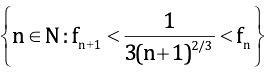then

1. a. A = N
2. b. A is a finite set
3. c. the complement of A in N is nonempty but finite
4. d. A and its complement in N are both infinite

Solution:

Given fn = (n + 1)1/3 – n1/3 ………….. (1)

Then fn+1 = (n + 2)1/3 – (n + 1)1/3 ………….. (2)

Now, Let, h(x) = x1/3 ; x ∈ R ………….. (3)

Plot graph of h(x) = x1/3

And let n, n + 1, n + 2 be the integer points on positive x-axis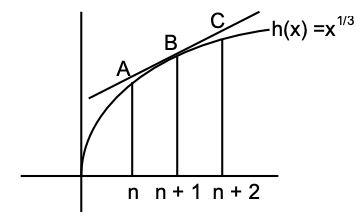Slope of BC = [h (n + 2) – h (n + 1)] / [(n + 2) - (n + 1)] {Formula of Slope of the line = [y2 - y1] / [x2 - x1]}

Slope of BC = [(n + 2) - (n + 1)] / 1 {from equation (3)}

Slope of BC = (n + 2)1/3 – (n + 1)1/3 = fn+ 1 {from equation (2)}

Similarly, slope of AB = [h (n + 1) – h(n)] / [(n + 1) – (n)] {from equation (3)}

Slope of AB = (n + 1)1/3 – n1/3 = fn {From equation (1)}

And slope of tangent to the curve h(x) = x1/3 at B

= (d / dx) (h (x)) at x = n + 1

= (1 / 3x2/3) at x = n + 1

= 1 / 3(n + 1)

Since curve is concave downward, slope is decreasing

Slope of BC < slope of tangent at B < slope of AB

fn + 1< 1 / 3(n + 1) < fn

This is true for all n ∈ N.

A = N

Question 19: A prime number p is called special if there exist prime p1, p2, p3, p4 such that p = p1 + p2 = p3 – p4. The number of special primes is

1. a. 0
2. b. 1
3. c. more than one but finite
4. d. infinite

Solution:

If none of P1, P2, P3, P4 is 2

All P1, P2, P3, P4 are odd P1 + P2 and P3 – P4 are both odd and hence cannot be prime.

One of P1 or P2 (say p1) and P4 must be 2

p = 2 + P2 = P3 – 2

Above equation is satisfied only if

P = 5, P2 = 3 and P3 = 7

So, only one possibility exists.

Question 20: Let ABC be a triangle in which AB = BC. Let X be a point on AB such that AX : XB = AB : AX. If AC = AX, then the measure of ∠ABC equals

1. a. 18°
2. b. 36°
3. c. 54°
4. d. 72°

Solution: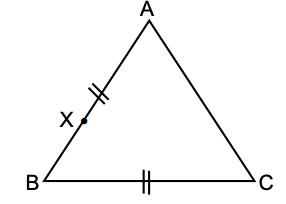Given AB = BC

AX : XB = AB : XA

AC = AX

Given that

AX:XB = AB : XA

AX/XB = AB/XA

AX/[XB + XA] = AB/[AX + AB] {Apply the componendo theorem}

AX/AB = AB/[AX + AB] {According to the diagram AB = XA + XB}

AX2 + AX × AB = AB2

Now let us add the term (AB / 2)2 on the both sides to make it look like the formula of expansion of the form (a + b)2 = a2 + b2 + 2ab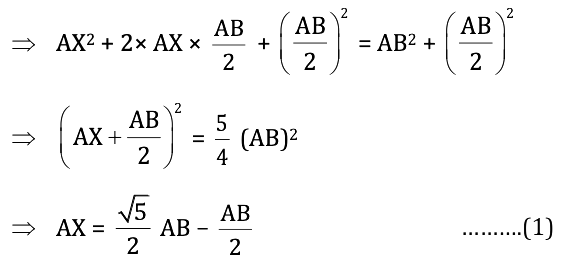Now let the side of the triangle AB = a

Given that AB = BC, therefore AB = BC = a

AC = AX = [(√5 - 1) / 2]a {from equation (1)}

Now in the triangle ABC

We know that cos θ = adjacent / hypotenuse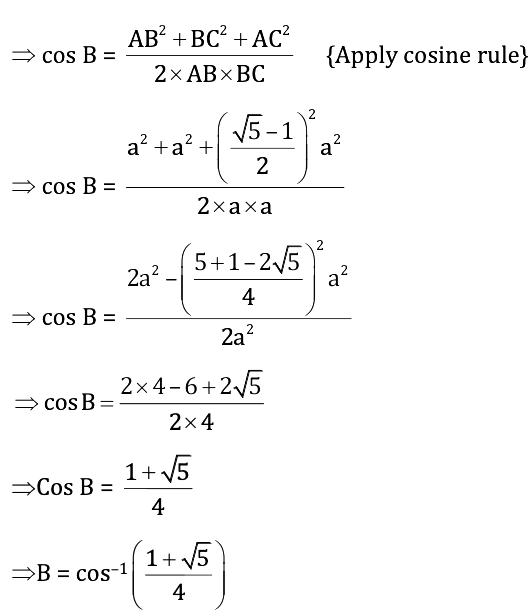B = 36°

ABC = 36°

Therefore the measure of ∠ABC is equal to 36°.

### KVPY SA 2019 Maths Paper with Solutions